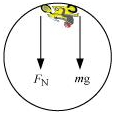## Pages

### Laws of motion NCERT Solutions Class 11 Physics - Solved Exercise Question 5.38

Question 5.38:
You may have seen in a circus a motorcyclist driving in vertical loops inside a ‘death-well’ (a hollow spherical chamber with holes, so the spectators can watch from outside). Explain clearly why the motorcyclist does not drop down when he is at the uppermost point, with no support from below. What is the minimum speed required at the uppermost position to perform a vertical loop if the radius of the chamber is 25 m?
Solution:
In a death-well, a motorcyclist does not fall at the top point of a vertical loop because both the force of normal reaction and the weight of the motorcyclist act downward and are balanced by the centripetal force. This situation is shown in the following figure.The net force acting on the motorcyclist is the sum of the normal force (FN) and the force due to gravity (Fg = mg).
The equation of motion for the centripetal acceleration ac, can be written as:
Fnet = mac
FN + Fg = mac
FN + mg = mv2 / r
Normal reaction is provided by the speed of the motorcyclist. At the minimum speed (vmin), FN = 0
mg = mvmin2 / r
∴ vmin = √rg

= √25 X 10 = 15.8 m/s